Download Presentation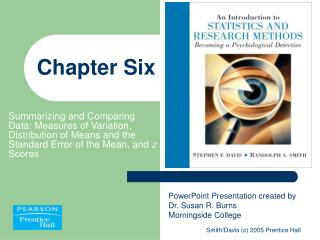Chapter Six

# Chapter Six - PowerPoint PPT Presentation

Chapter Six. Summarizing and Comparing Data: Measures of Variation, Distribution of Means and the Standard Error of the Mean, and z Scores. PowerPoint Presentation created by Dr. Susan R. Burns Morningside College . Measures of Variability. Variability indicates the spread in scores.I am the owner, or an agent authorized to act on behalf of the owner, of the copyrighted work described.
Download Presentation## Chapter Six

An Image/Link below is provided (as is) to download presentation

Download Policy: Content on the Website is provided to you AS IS for your information and personal use and may not be sold / licensed / shared on other websites without getting consent from its author.While downloading, if for some reason you are not able to download a presentation, the publisher may have deleted the file from their server.

- - - - - - - - - - - - - - - - - - - - - - - - - - E N D - - - - - - - - - - - - - - - - - - - - - - - - - -
Presentation Transcript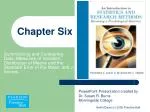### Chapter Six

Summarizing and Comparing Data: Measures of Variation, Distribution of Means and the Standard Error of the Mean, and z Scores

PowerPoint Presentation created by Dr. Susan R. BurnsMorningside College

Smith/Davis (c) 2005 Prentice Hall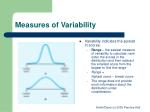Measures of Variability
• Variability indicates the spread in scores.
• Range – the easiest measure of variability to calculate; rank order the scores in the distribution and then subtract the smallest score from the largest to find the range
• Range =

highest score – lowest score

• The range does not provide much information about the distribution under consideration.

Smith/Davis (c) 2005 Prentice Hall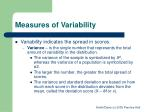Measures of Variability
• Variability indicates the spread in scores.
• Variance – is the single number that represents the total amount of variability in the distribution.
• The variance of the sample is symbolized by S2, whereas the variance of a population is symbolized by σ2.
• The larger the number, the greater the total spread of scores.
• The variance and standard deviation are based on how much each score in the distribution deviates from the mean, called the deviation score (X – M or x).

Smith/Davis (c) 2005 Prentice Hall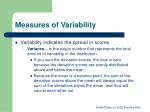Measures of Variability
• Variability indicates the spread in scores.
• Variance – is the single number that represents the total amount of variability in the distribution.
• If you sum the deviation scores, the total is zero because the deviation scores are evenly distributed above and below the mean.
• Because the mean is a balance point, the sum of the deviation scores above the mean will always equal the sum of the deviations below the mean, and thus will cancel each other out.

Smith/Davis (c) 2005 Prentice Hall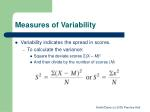Measures of Variability
• Variability indicates the spread in scores.
• To calculate the variance:
• Square the deviate scores Σ(X – M)2
• And then divide by the number of scores (N)

Smith/Davis (c) 2005 Prentice Hall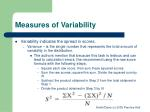Measures of Variability
• Variability indicates the spread in scores.
• Variance – is the single number that represents the total amount of variability in the distribution.
• The authors mention that because this task is tedious and can lead to calculation errors, the recommend using the raw score formula with the following steps:
• Square each raw score, then sum these squared scores
• Sum the raw scores; square this sum. Divide the squared sum by N.
• Subtract the product obtained in Step 2 from the total obtained in Step 1.
• Divide the product obtained in Step 3 by N.

Smith/Davis (c) 2005 Prentice Hall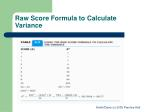Raw Score Formula to Calculate Variance

Smith/Davis (c) 2005 Prentice Hall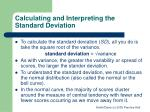Calculating and Interpreting the Standard Deviation
• To calculate the standard deviation (SD), all you do is take the square root of the variance.

standard deviation = √variance

• As with variance, the greater the variability or spread of scores, the larger the stander deviation.
• To understand the standard deviation, we must discuss the normal distribution (also called the normal or the bell curve).
• In the normal curve, the majority of scores cluster around the measure of central tendency with fewer and fewer scores occurring as we move away from it.

Smith/Davis (c) 2005 Prentice Hall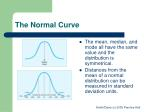The Normal Curve
• The mean, median, and mode all have the same value and the distribution is symmetrical.
• Distances from the mean of a normal distribution can be measured in standard deviation units.

Smith/Davis (c) 2005 Prentice Hall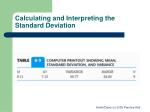Calculating and Interpreting the Standard Deviation

Smith/Davis (c) 2005 Prentice HallCalculating and Interpreting the Standard Deviation
• When we describe the shape of a normal distribution, how flat or peaked it is describes its kurtosis. There are three types of kurtosis:
• Leptokurtic distributions are tall and peaked. Because the scores are clustered around the mean, the standard deviation will be smaller.
• Mesokurtic distributionsare the ideal example of the normal distribution, somewhere between the leptokurtic and playtykurtic.
• Platykurtic distributions are broad and flat.

Smith/Davis (c) 2005 Prentice HallDistribution of Means and the Standard Error of the Mean
• A distribution of means is where the “scores” in the distribution are means, not scores from individual participants with the following characteristics:
• The distribution of means will approximate a normal distribution if the population is a normal distribution or if each sample you randomly select contains at least 30 scores. If the distribution of means is a normal distribution, then we already have considerable information concerning the percentage of scores falling the respective standard deviations.

Smith/Davis (c) 2005 Prentice HallDistribution of Means and the Standard Error of the Mean
• A distribution of means is where the “scores” in the distribution are means, not scores from individual participants with the following characteristics:
• The mean of a distribution of means will be the same as the mean of the population. Using the Greek letter mu, μ, to symbolize the mean of a population and μM to symbolize the mean of a distribution of means, we can say that μM = μ.
• You can calculate the variance for a distribution of means; you simply treat each mean as a raw score and proceed as you would in calculating any variance. Once you have calculated the variance of a distribution of means, it is interesting to note that this variance is equal to the population variance divided by the number in each of your samples with the following formula:

Smith/Davis (c) 2005 Prentice HallDistribution of Means and the Standard Error of the Mean
• A distribution of means is where the “scores” in the distribution are means, not scores from individual participants with the following characteristics:
• The mean of a distribution of means will be the same as the mean of the population. Using the Greek letter mu, μ, to symbolize the mean of a population and μM to symbolize the mean of a distribution of means, we can say that μM = μ.
• As with any other variance, the standard deviation of a distribution of means is the square root of the variance of the distribution of means with the following formulas:

Smith/Davis (c) 2005 Prentice HallDistribution of Means and the Standard Error of the Mean
• A distribution of means is where the “scores” in the distribution are means, not scores from individual participants with the following characteristics:
• The standard deviation of a distribution of means is known as the standard error of the mean (SEM), which tells you how much variability you will find in the population from which you drew the sample(s) in your research.

Smith/Davis (c) 2005 Prentice Hallz Scores
• z scores provide the distance, in standard deviation units, of a raw score from the mean.
• The formula for a z Scores is as follows:
• To convert a z score back to a raw score you can use the following formula:

X = (z)(SD) + M

Smith/Davis (c) 2005 Prentice HallConfidence Intervals
• Statisticians have combined their knowledge of the normal curve and an interest in the distributions of sample means to produce another important concept: confidence intervals
• Interval estimates – are estimates of the range or interval that is likely to include the population characteristic (called a parameter), such as the mean or variance. An interval that has a specific percentage associated with it is called a confidence interval.
• For the 95% confidence interval we are seeking limits that will encompass a total of 95%, 47.5% above the mean, 47.5% below the mean.
• The 99% confidence interval encompasses a total of 99%, 49.5% above and 49.5% below the mean.

Smith/Davis (c) 2005 Prentice HallConfidence Intervals
• Calculation of the two confidence intervals consists of:
• 95% Confidence Interval
• Multiply SD by –z score (-1.96) and +z score (+1.96)
• Subtract the first product from the mean to establish the lower confidence limit.
• Add the second product to the mean to establish the upper confidence limit.
• 99% Confidence Interval
• Multiply SD by –z score (-2.57) and +z score (+2.57)
• Subtract the first product from the mean to establish the lower confidence limit.
• Add the second product to the mean to establish the upper confidence limit.

Smith/Davis (c) 2005 Prentice Hall